# 1. Functional Regression

Let the covariatebe an at least twice continuously differentiable random function defined wlog. on an intervalandthe corresponding the response. For simplicity we assume centered random variables, i.e.beside we require bounded second moments,. We assume that there exists somethat satisfies

(1)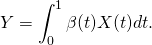We define cross-covariance functions of X and Y given by

(2)Letbe the covariance operator ofas defined in this post with ordered eigenvalues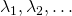and corresponding eigenfunctions, then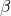statisfies (1) if and only if

(3)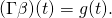(3) has a solution if and only ifis invertible, forthe unique solution is then given by

(4)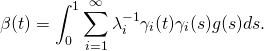However this solution is unstable as to be seen by a simple example. Consider some, and define. Then as(5)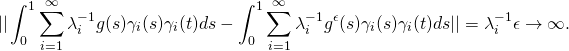This means that small pertubations inleads to a large variation (even infinite) in. There are various ways to tackle this problem, the key idea is to trade the uniqness for the stabilty of the a solution. This is archieved by reducing the dimensionality of the covariance operator. By doing so we do not solve the same problem but another one that is somewhat close to the original problem.
A popular approach is to smooth the regression or to truncate the expansion after some. This truncation aproach will be the foundation of our spark implementation.

## 1.1 Simulation

For our simulation we will again assume a brownian motiononand. For the simulation we sample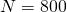curves and approximateas proposed in this post with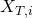attimepoints, accordingly.

## 1.2 Implementation in Spark

Our implementation is based on the FPCA Algorithm presented in this post. We use the estimated firsteigenfunctionsand corresponding eigenvaluesto construct an estimator based on 5 forfor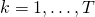with#We start with a sample of brownian motions
import matplotlib.pyplot as plt
from numpy import *
from pyspark.mllib.linalg import *
from pyspark.mllib.linalg.distributed import *
from pyspark.mllib.linalg.distributed import CoordinateMatrix, MatrixEntry

def spark_FPCA( matrix , L):
N = matrix.numRows()
T = matrix.numCols()
scale =CoordinateMatrix(sc.parallelize(range(N)).map(lambda i: MatrixEntry(i, i, 1./sqrt(T) )), N, N).toBlockMatrix()
# Compute the top L singular values and corresponding singular vectors.
svd = scale.multiply(matrix).transpose().toIndexedRowMatrix().computeSVD(L)
s = svd.s       # The singular values are stored in a local dense vector.
Va=svd.V.toArray()
Vl = list();
for i in range(0, len(Va)):
Vl.append( IndexedRow(i, Va[i] ))
V=IndexedRowMatrix( sc.parallelize(Vl) )
S=DenseMatrix( L,L, diag(s).flatten().flatten().tolist() )
Si=DenseMatrix( L,L, diag(1./(s)).flatten().flatten().tolist() )
scores=V.multiply(S)
components= matrix.transpose().multiply( V.multiply(Si).toBlockMatrix() )
#reduced FPCA decomposition
FPCA= components.multiply(scores.toBlockMatrix().transpose() )
return (components, scores, FPCA, s)

def spark_FReg( Xmatrix ,Ymatrix , L):
fpca=spark_FPCA( Xmatrix , L)
T = matrix.numCols()
components=fpca
s=fpca*fpca
#reconstruct beta
Si=DenseMatrix( L,L, diag(1./(s*T)).flatten().flatten().tolist() )
beta_est=Ymatrix.transpose().multiply( Xmatrix ).multiply(components).toIndexedRowMatrix().multiply(Si).toBlockMatrix().multiply(components.transpose())
return (beta_est)

##EXAMPLE
T=5000
N=800

#define beta(t), the score function
time=2*pi*true_divide(arange(0,T),T )
beta= sin( time)
#betamat=DenseMatrix( T,N, np.repeat(beta,N) )
betamat=DenseMatrix( T,1, beta )
scaleMat=DenseMatrix( T,T, diag(1/T).flatten().flatten().tolist() )

#plt.plot(time,beta)
#plt.show()

data = list();
for i in range(0, N):
data.append( IndexedRow(i, array( cumsum(random.normal(0,sqrt(true_divide(1,T)),T)) ) ) )

#convert the data to spark
sc_data= sc.parallelize( data )
matrix = IndexedRowMatrix(sc_data).toBlockMatrix().cache()

scale=CoordinateMatrix(sc.range(N).map(lambda i: MatrixEntry(i, i, 1./T)), N, N).toBlockMatrix()
Ymatrix=scale.multiply(IndexedRowMatrix(sc_data).multiply(betamat).toBlockMatrix()).cache()

##Plot the Input data
#plt.plot( Ymatrix.toLocalMatrix().toArray().flatten() )
#plt.show()

#reconstruct beta
beta_est=spark_FReg(matrix, Ymatrix, 12).toLocalMatrix()

times=true_divide( arange(0,T),T )
plt.plot(times, beta_est.toArray().flatten())

plt.plot(times,beta )
plt.show()


This site uses Akismet to reduce spam. Learn how your comment data is processed.